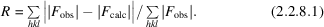International
Tables for
Crystallography
Volume F
Crystallography of biological macromolecules
Edited by E. Arnold, D. M. Himmel and M. G. Rossmann

International Tables for Crystallography (2012). Vol. F, ch. 2.2, p. 71

## Section 2.2.8. Quality indicators for refinement

H. M. Einspahra* and M. S. Weissb

aPO Box 6483, Lawrenceville, NJ 08648–0483, United States, and bHelmholtz-Zentrum Berlin für Materialien und Energie, Macromolecular Crystallography (HZB-MX), Albert-Einstein-Str. 15, D-12489 Berlin, Germany
Correspondence e-mail:  hmeinspahr@yahoo.com

### 2.2.8. Quality indicators for refinement

| top | pdf |

The last step of a structure determination is the refinement of the model against the observed data. Refinement is in principle a mathematical operation that is applied in order to minimize the discrepancy between the observed structure-factor amplitudes |Fobs| and the calculated ones |Fcalc|.

Crystallographic R factor, R. The crystallographic R factor R is defined as the fractional disagreement between the set of observed structure-factor amplitudes and amplitudes calculated from the structural model. Of course, observed and calculated reflection sets need to be on the same scale.Free R factor, Rfree. The free R factor Rfree is defined in the same way as the crystallographic R factor, but it is based on a set of reflections that have been excluded from the refinement (Brünger, 1992). The excluded set of reflections is called the test set, while the set of reflections used for refinement is called the working set. The test set can be chosen randomly or systematically, either in thin resolution shells or to account for the presence of noncrystallographic symmetry, respectively. In order to minimize the impact on the final model, the test set should be as small as possible. Typically, it contains about 5–10% of the reflections, or at least enough reflections to keep the standard deviation of Rfree below 1%, but there is no need to use more than 2000 reflections (Kleywegt & Brünger, 1996; Brünger, 1997). The standard deviation of Rfree has been empirically estimated to be Rfree/N1/2, where N is the number of reflections in the test set (Brünger, 1997). Of course, there may be concerns about the impact of excluding 5–10% of reflections on the final model, but a few final cycles of refinement against the recombined full data set should allay them.

Correlation coefficients CC(Fobs, Fcalc) and CC(Iobs, Icalc). CC(Fobs, Fcalc) and CC(Iobs, Icalc) are Pearson linear correlation coefficients [see equation (2.2.2.13)] between observed and model-based calculated structure-factor amplitudes or intensities, respectively, that find use from time to time. One advantage of the use of a correlation coefficient instead of an R factor is that it avoids the problem of scaling the two sets of numbers relative to each other.

### References

Brünger, A. T. (1992). Free R value: a novel statistical quantity for assessing the accuracy of crystal structures. Nature (London), 355, 472–475.
Brünger, A. T. (1997). Free R value: cross-validation in crystallography. Methods Enzymol. 277, 366–396.
Kleywegt, G. & Brünger, A. T. (1996). Checking your imagination: applications of the free R value. Structure, 4, 897–904.